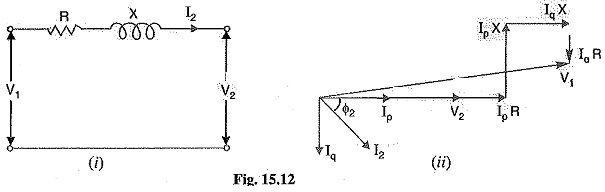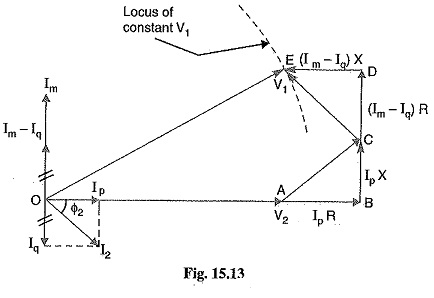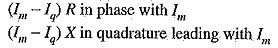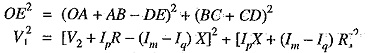## Voltage Control by Synchronous Condenser:

The voltage at the receiving end of a transmission line can be controlled by installing specially designed synchronous motors called synchronous condensers at the receiving end of the line. The Voltage Control by Synchronous Condenser supplies wattless leading kVA to the line depending upon the excitation of the motor. This wattless leading kVA partly or fully cancels the wattless lagging kVA of the line, thus controlling the voltage drop in the line. In this way, voltage at the receiving end of a transmission line can be kept constant as the load on the, system changes.

For simplicity, consider a short transmission line where the effects of capacitance are neglected. Therefore, the line has only resistance and inductance. Let V1 and V2 be the per phase sending end and receiving end voltages respectively. Let I2 be the load current at a lagging power factor of cos Φ2.

1.Without synchronous condenser: Fig. 15.12 (0 shows the transmission line with resis­tance R and inductive reactance X per phase. The load current I2 can be resolved into two rectangular components viz Ip in phase with V2 and Iq at right angles to V2 [See Fig. 15.12 (ii)]. Each component will produce resistive and reactive drops ; the resistive drops being in phase with and the reactive drops in quadrature leading with the corresponding currents. The vector addition of these voltage drops to V2 gives the sending end voltage V1.2.With synchronous condenser: Now suppose that a synchronous condenser taking a lead­ing current Im is connected at the receiving end of the line. The vector diagram of the circuit becomes as shown in Fig. 15.13. Note that since Im and Iq are in direct opposition and that Im must be greater than Iq, the four drops due to these two currents simplify to :From the vector diagram, the relation between V1 and V2 is given byFrom this equation, the value of Im can be calculated to obtain any desired ratio of V1/V2 for a given load current and power factor.

Scroll to Top